### Introduction

Textbook: Artificial Intelligence: A modern Approach, Stuart Russell and Peter Nerving, 3rd Edition, 2009

Reference: CS188, Berkeley

• Symbolism
• Statistical Approaches
• Connectionism

Trends: Three types of approaches will be integrated.

Reflex Agents: consider how the world IS, decisions based on current percept(memory)
Planning Agents: consider how the world WOULD BE, decisions based on consequences of actions

Search Problem:
Def:
1.A state space
2.A successor function
3.Start / Goal state
Methods:
State space Graphs
Search Trees
Properties:
Complete? (find a solution if exists)
Optimal? (find the least cost path)
Time complexity
Space complexity

#### Depth-first Search (DFS)

strategy: expand a deepest node first
Stack: last in first out
Complete only if no circles.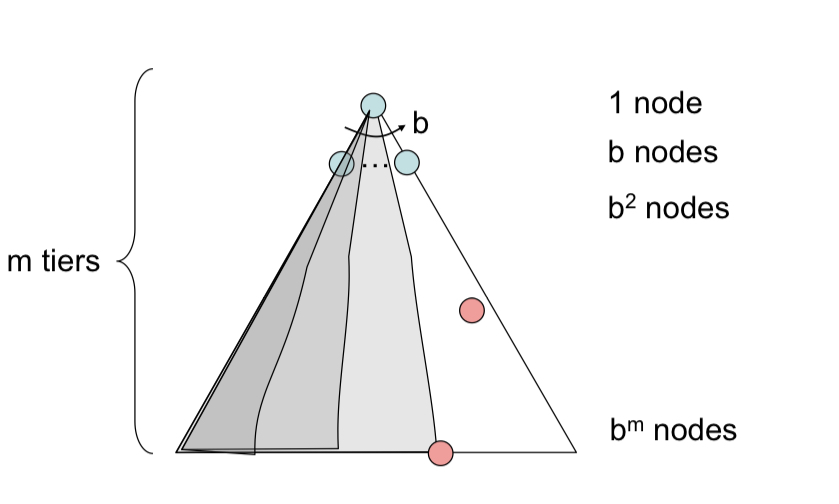#### Breadth-first Searc (BFS)

strategy: expand a shallowest node first
Queue: first in first out
Optimal only if all costs are 1, because it finds the shortest path.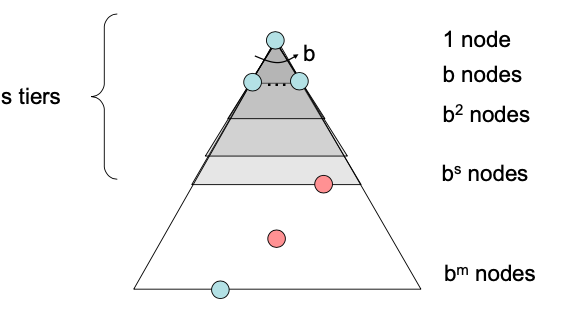#### Iterative Deepening

DFS space + BFS time
strategy: run a DFS with depth limit 1, 2, 3…

#### Uniform Cost Search (UCS)

strategy: expand the cheapest node first
Priority Queue (priority: cumulative cost)

Complete Optimal Time Space Fringe
DFS x x $O(b^m)$ $O(bm)$ Stack
BFS $\sqrt{}$ x $O(b^s)$ $O(b^s)$ Queue
UCS $\sqrt{}$ $\sqrt{}$ $O(b^{C^*/\epsilon})$ $O(b^{C^*/\epsilon})$ Priority Queue
$A^*$ $\sqrt{}$ x

Heuristics $h$: estimate how close to a goal
Example: Manhattan distance, Euclidean distance
strategy: expand the node that is “closest” to goal

UCS (g) + Greedy (h)
$f(n) = g(n) + (n)$

A $h$ is admissible(optimistic) if: $0 \leq h(n) \leq h^{*}(n)$
where $h^*(n)$ is the true cost to a nearest goal.

Optimality of A* search:
Assume: $A$ is an optimal goal node, $B$ is a suboptimal goal node, $h$ is admissible. Then $A$ will exit the fringe before $B$.

Proof: Imagine $B$ is on the fringe, some ancestor $n$ of $A$ is on the fringe, too. We need to show that $n$ will be expanded before $B$.

All ancestors of A expand before B, A expands before B, A* search is optimal.

Idea: never expand a state twice

Method: tree search + closed set (set of expanded states)

Admissibility: heuristic cost $\leq$ actual cost to goal, $h(A)\leq \text{cost(A to G)}$
Consistency: heuristic arc cost $\leq$ actual cost for each arc, $h(A) - h(C)\leq \text{cost(A to C)}$
Consistency example: manhattan, euclidean distance

Optimal of A Tree search: admissibility
Optimal of A
Graph search: consistency

### Constraint Satisfaction Problems (CSP)

Search Basics: Backtracking Search
DFS. One variable at a time, check constraints as you go.

#### Filtering

Filtering: Keep track of domains for unassigned variables and cross off bad options.

Forward checking:
Cross off values that violate a constraint when added to the existing assignment; whenever any variable has no value left, we backtrack.
propagates information from assigned to unassigned variables, but doesn’t provide early detection for all failures.

Arc Consistency:
Def: X $\rightarrow$ Y is consistent, if every tail x there is a y in the head.
If not consistent, delete from the tail.
If head Y loses a value, then arc needs to be rechecked.
Backtracking + arc consistency

K-consistency:
k = 1: node consistency;
k = 2: arc consistency;
k = 3: path consistency, if binary constraint networks;
Strong k-consistency: also k-1, k-2, … 1 consistent.
Strong k-consistency means that we can find the solution without backtracing.

#### Ordering

Variable Ordering
Minimum remaining values (MRV): choose the variable with the fewest legal left values in its domain.

Value Ordering:
Least constraining values: choose the one that rules out the fewest values in the remaining variables.

#### Structure

Independent Subproblems:
Independent subproblems are identifiable as connected components of constraint graph.
Suppose a problem with $n$ variables can be broken into subprobelms of only $c$ variables.

Time cost from expo to linear.

Tree structure:
If the constraint graph has no loop, then time complexity $O(d^2n)$.
There is an arc between each two nodes, each of which must compare up to $d$ possible domain values for two variables. $O(d^2n)$, which is linear.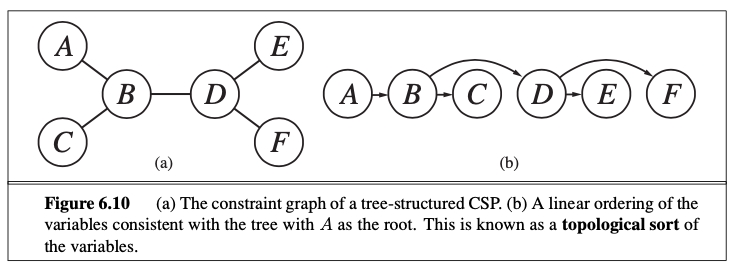Cutset Conditioning:

#### Minimax

In deterministic, zero-sum games:
One player maximizes result, the other minimizes.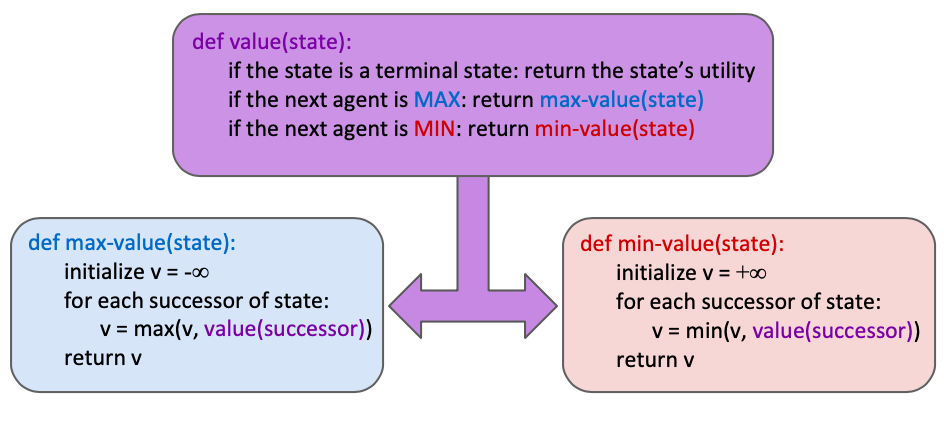The recursion proceeds all the way down to the leaves of the tree, and then minimax values are backed up through the tree as the recursion unwinds.
Performs a complete depth-first exploration. Time $O(b^m)$, space $O(bm)$. Problem: infeasible usually. Need improvement.

Depth-limited Search:
Search limited depth in a tree. Replace terminal utilities with an evaluation function.

Evaluation Funtions:
$Eval(s) = w_1f_1(s) + w_2f_2(s) + \cdots w_nf_n(s)$
Monte Carlo tree search
Convolutional neural network

Minimax Pruning*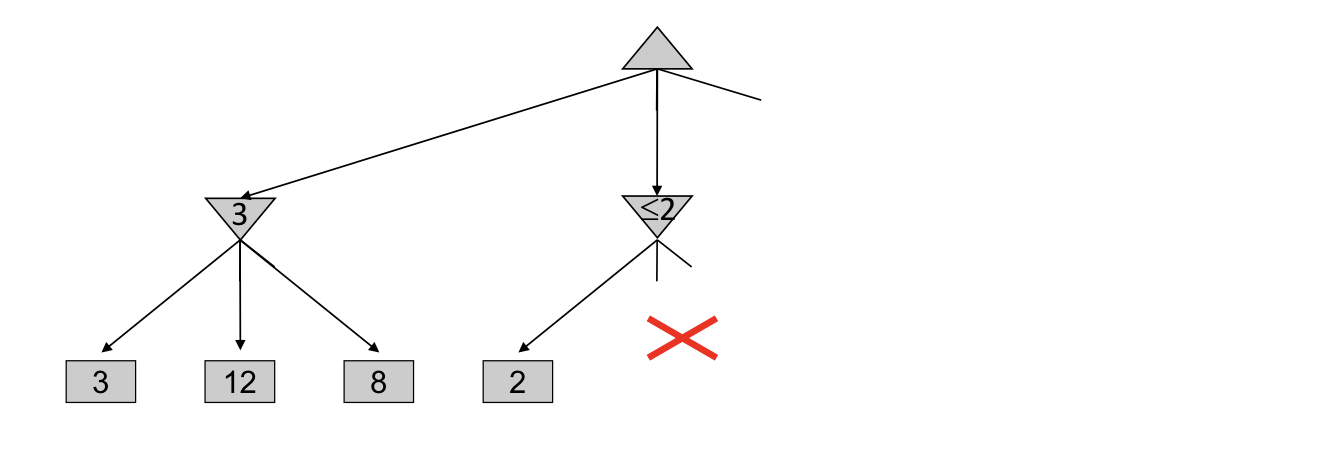#### Alpha-Beta Pruning

Consider some node $n$ in the tree, if the player has better choice $m$ at the ancestor of node $n$, then $n$ will never be reached in actual play.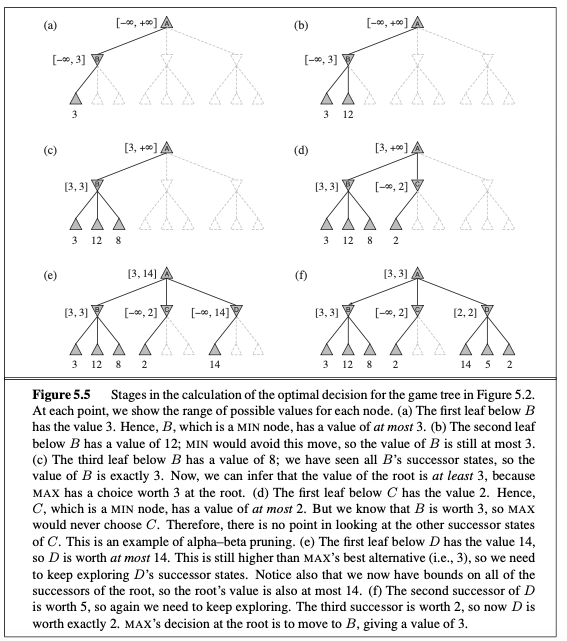$\alpha$ = highest value so far for MAX
$\beta$ = lowest value so far so MIN

### Bayes Nets: Exact Inference

#### Inference by Enumeration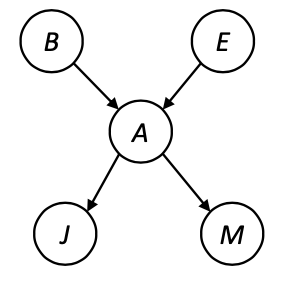#### Factors

Factor: multi-dimensional array

Join Factors: $\qquad \forall r,t:\quad P(r,t)=P(r)\cdot P(t|r)$

Eliminate: $\qquad \text{Sum }R, \quad P(R,T)\Rightarrow P(T)$